# 18.8 Thiols and Sulfides

Objectives

After completing this section, you should be able to

1. write the IUPAC name of a thiol, given its Kekulé, condensed or shorthand structure.
2. draw the structure of a thiol, given its IUPAC name.
3. write an equation to represent the formation of a thiol by the reaction of hydrosulfide anion with an alkyl halide.
4. write an equation to illustrate the preparation of a thiol by the reaction of thiourea with an alkyl halide.
1. write an equation to show the interconversion between thiols and disulfides.
1. write the name of a sulfide, given its structure.
2. draw the structure of a sulfide, given its name.
3. write an equation showing how a sulfide may be prepared by the reaction of a thiolate anion on an alkyl halide.
4. identify the product from the reaction of a given alkyl halide with a given thiolate anion.
5. identify the reagents necessary to prepare a given sulfide.
6. write an equation to illustrate the formation of a trialkylsulfonium salt from a sulfide and an alkyl halide.

Key Terms

Note: All of these terms are defined in the “Study Notes,” below.

• disulfide
• mercapto group
• (organic) sulfide
• sulfone
• sulfoxide
• thiol
• thiolate anion
• trialkylsulfonium ion (trialkylsulfonium salt)

Study Notes

The chemistry of sulfur‑containing organic compounds is often omitted from introductory organic chemistry courses. However, we have included a short section on these compounds, not for the sake of increasing the amount of material to be digested, but because much of the chemistry of these substances can be predicted from a knowledge of their oxygen‑containing analogues.

A thiol is a compound which contains an $\ce{-}$SH functional group. The $\ce{-}$SH group itself is called a mercapto group. A disulfide is a compound containing an $\ce{-}$S$\ce{-}$S$\ce{-}$ linkage.

(Organic) sulfides have the structure R$\ce{-}$S$\ce{-}$R′, and are therefore the sulfur analogues of ethers. The nomenclature of sulfides can be easily understood if one understands the nomenclature of the corresponding ethers. Notice that the term “thio” is also used in inorganic chemistry. For example, SO42− is the sulfate ion; while S2O32−, in which one of the oxygen atoms of a sulfate ion has been replaced by a sulfur atom, is called thiosulfate.

Thiolate anions, RS$\ce{-}$, are analogous to alkoxy anions, RO$\ce{-}$. Thiolate anions are better nucleophiles than are alkoxy anions.

If you have trouble understanding why trialkylsulfonium ions are formed, think of them as being somewhat similar to the hydronium ions that are formed by protonating water:Later we shall see examples of tetraalkylammonium ions, R4N+, which again may be regarded as being similar to hydronium ions.

Sulfoxides and sulfones are obtained by oxidizing organic sulfides. You need not memorize the methods used to carry out these oxidations.Table 18.1, below, provides a quick comparison of oxygen‑containing and sulfur‑containing organic compounds.

Oxygen‑containing Compound Sulfur Analogue
ether, R$\ce{-}$O$\ce{-}$R′ sulfide, R$\ce{-}$S$\ce{-}$R′
R$\ce{-}$O$\ce{-}$, alkoxy group R$\ce{-}$S$\ce{-}$, alkylthio group
R$\ce{-}$O, alkoxy anion R$\ce{-}$S, thiolate anion
alcohol, R$\ce{-}$OH thiol, R$\ce{-}$SH
$\ce{-}$OH, hydroxy group $\ce{-}$SH, mercapto group
R$\ce{-}$O$\ce{-}$O$\ce{-}$R′, peroxide R$\ce{-}$S$\ce{-}$S$\ce{-}$R′, disulfide

Table 18.1 Comparison of compounds containing oxygen and sulfur

Note that when we name thiols, we include the “e” of the alkane name. Thus, CH3CH2SH is called “ethanethiol,” not “ethanthiol.”

## Oxidation States of Sulfur CompoundsOxygen assumes only two oxidation states in its organic compounds (–1 in peroxides and –2 in other compounds). Sulfur, on the other hand, is found in oxidation states ranging from –2 to +6, as shown in the following table (some simple inorganic compounds are displayed in orange).## Thiols

Thiols, which are also called mercaptans, are analogous to alcohols. They are named in a similar fashion as alcohols except the suffix -thiol is used in place of -ol. By itself the -SH group is called a mercapto group.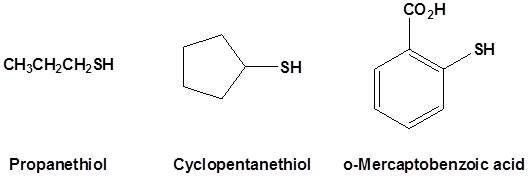Thiols are usually prepared by using the hydrosulfide anion (-SH) as a neucleophile in an SN2 reaction with alkyl halides.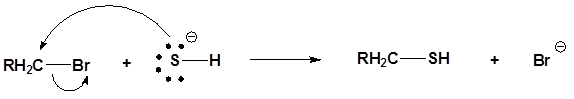On problem with this reaction is that the thiol product can undergo a second SN2 reaction with an additional alkyl halide to produce a sulfide side product. This problem can be solved by using thiourea, (NH2)2C=S, as the nucleophile. The reaction first produces an alkyl isothiourea salt and an intermediate. This salt is then hydrolyzed by a reaction with aqueous base.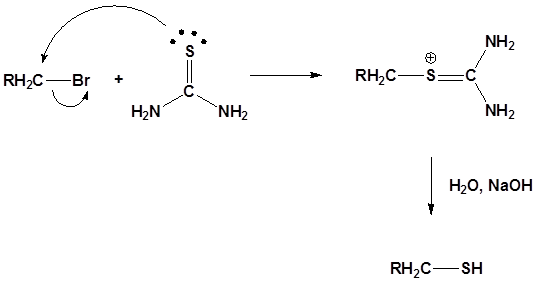## Disulfides

Oxidation of thiols and other sulfur compounds changes the oxidation state of sulfur rather than carbon. We see some representative sulfur oxidations in the following examples. In the first case, mild oxidation converts thiols to disufides. An equivalent oxidation of alcohols to peroxides is not normally observed. The reasons for this different behavior are not hard to identify. The S–S single bond is nearly twice as strong as the O–O bond in peroxides, and the O–H bond is more than 25 kcal/mole stronger than an S–H bond. Thus, thermodynamics favors disulfide formation over peroxide.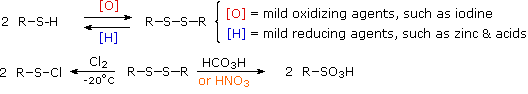## Disulfide bridges in proteinsDisulfide (sulfur-sulfur) linkages between two cysteine residues are an integral component of the three-dimensional structure of many proteins. The interconversion between thiols and disulfide groups is a redox reaction: the thiol is the reduced state, and the disulfide is the oxidized state.Notice that in the oxidized (disulfide) state, each sulfur atom has lost a bond to hydrogen and gained a bond to a sulfur - this is why the disulfide state is considered to be oxidized relative to the thiol state. The redox agent that mediates the formation and degradation of disulfide bridges in most proteins is glutathione, a versatile coenzyme that we have met before in a different context (section 14.2A). Recall that the important functional group in glutathione is the thiol, highlighted in blue in the figure below. In its reduced (free thiol) form, glutathione is abbreviated 'GSH'.In its oxidized form, glutathione exists as a dimer of two molecules linked by a disulfide group, and is abbreviated 'GSSG'.

A new disulfide in a protein forms via a 'disulfide exchange' reaction with GSSH, a process that can be described as a combination of two SN2-like attacks. The end result is that a new cysteine-cysteine disulfide forms at the expense of the disulfide in GSSG.In its reduced (thiol) state, glutathione can reduce disulfides bridges in proteins through the reverse of the above reaction.

Disulfide bridges exist for the most part only in proteins that are located outside the cell. Inside the cell, cysteines are kept in their reduced (free thiol) state by a high intracellular concentration of GSH, which in turn is kept in a reduced state (ie. GSH rather than GSSG) by a flavin-dependent enzyme called glutathione reductase.

Disulfide bridges in proteins can also be directly reduced by another flavin-dependent enzyme called 'thioredoxin'. In both cases, NADPH is the ultimate electron donor, reducing FAD back to FADH2 in each catalytic cycle.

In the biochemistry lab, proteins are often maintained in their reduced (free thiol) state by incubation in buffer containing an excess concentration of b-mercaptoethanol (BME) or dithiothreitol (DTT). These reducing agents function in a manner similar to that of GSH, except that DTT, because it has two thiol groups, forms an intramolecular disulfide in its oxidized form.## Sulfides

Sulfur analogs of ethers are called sulfides. The chemical behavior of sulfides contrasts with that of ethers in some important ways. Since hydrogen sulfide (H2S) is a much stronger acid than water (by more than ten million fold), we expect, and find, thiols to be stronger acids than equivalent alcohols and phenols. Thiolate conjugate bases are easily formed, and have proven to be excellent nucleophiles in SN2 reactions of alkyl halides and tosylates.

R–S(–) Na(+) + (CH3)2CH–Br(CH3)2CH–S–R + Na(+) Br(–)

Although the basicity of ethers is roughly a hundred times greater than that of equivalent sulfides, the nucleophilicity of sulfur is much greater than that of oxygen, leading to a number of interesting and useful electrophilic substitutions of sulfur that are not normally observed for oxygen. Sulfides, for example, react with alkyl halides to give ternary sulfonium salts (equation # 1) in the same manner that 3º-amines are alkylated to quaternary ammonium salts. Although equivalent oxonium salts of ethers are known, they are only prepared under extreme conditions, and are exceptionally reactive.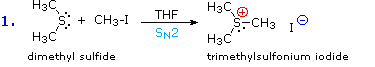sulfides are named using the same rules as ethers except sulfide is used in the place of ether. For more complex substance alkylthio is used instead of alkoxy.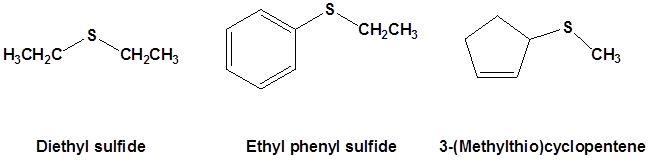### SAM methyltransferasesThe most common example of sulfonium ions in a living organism is the reaction of S-Adenosylmethionine. Some of the most important examples of SN2 reactions in biochemistry are those catalyzed by S-adenosyl methionine (SAM) – dependent methyltransferase enzymes. We have already seen, in chapter 6 and again in chapter 8, how a methyl group is transferred in an SN2 reaction from SAM to the amine group on the nucleotide base adenosine:Another SAM-dependent methylation reaction is catalyzed by an enzyme called catechol-O-methyltransferase. The substrate here is epinephrine, also known as adrenaline.Notice that in this example, the attacking nucleophile is an alcohol rather than an amine (that’s why the enzyme is called an O-methyltransferase). In both cases, though, a basic amino acid side chain is positioned in the active site in just the right place to deprotonate the nucleophilic group as it attacks, increasing its nucleophilicity. The electrophile in both reactions is a methyl carbon, so there is little steric hindrance to slow down the nucleophilic attack. The methyl carbon is electrophilic because it is bonded to a positively-charged sulfur, which is a powerful electron withdrawing group. The positive charge on the sulfur also makes it an excellent leaving group, as the resulting product will be a neutral and very stable sulfide. All in all, in both reactions we have a reasonably good nucleophile, an electron-poor, unhindered electrophile, and an excellent leaving group.

Because the electrophilic carbon in these reactions is a methyl carbon, a stepwise SN1-like mechanism is extremely unlikely: a methyl carbocation is very high in energy and thus is not a reasonable intermediate to propose. We can confidently predict that this reaction is SN2. Does this SN2 reaction occur, as expected, with inversion of stereochemistry? Of course, the electrophilic methyl carbon in these reactions is achiral, so inversion is not apparent. To demonstrate inversion, the following experiment has been carried out with catechol-O-methyltransferase:Here, the methyl group of SAM was made to be chiral by incorporating hydrogen isotopes tritium (3H, T) and deuterium (2H, D). The researchers determined that the reaction occurred with inversion of configuration, as expected for an SN2 displacement (J. Biol. Chem. 1980, 255, 9124).

Sulfides can be easily oxidized. Reacting a sulfide with hydrogen peroxide, H2O2, as room termpeature produces a sulfoxide (R2SO). The oxidation can be continued by reaction with a peroxyacid to produce the sulfone (R2SO2)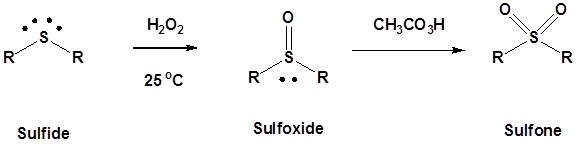A common example of a sulfoxide is the solvent dimethyl sulfoxide (DMSO). DMSO is polar aprotic solvent.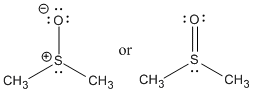Figure AB16.3. DMSO is a very polar, aprotic solvent.